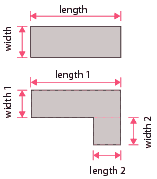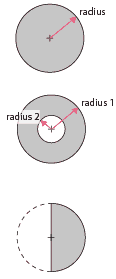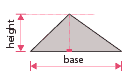home » general building » concrete volume calculator (imperial)

# Quantity/Volume Calculators for Concrete (Imperial)

These calculators are intended to allow you to calculate the imperial volume of concrete required for paths etc.

Three calculators are provided, the first for rectangle areas of concrete, the second for circular areas and the final one for triangular areas.

## Rectangular volume calculatorFor a simple rectangle, just use the length and width with the thickness in the calculator left.

For irregular, basically square areas,

• Breakdown the area into a number of simple rectangular areas.
• Workout the volume for each area separately.
• And finally add the separate volumes together.
 Enter the dimensions of the rectangle to be concreted: Length feet Width feet Tickness inches You will need approximately..... yds³ of concrete   Comment Note: This is the volume of the work space and does not take into account the concrete 'shrinkage'

## Circular volume calculatorFor triangular shapes, work out the height and base of the triangle and use with the thickness in the calculator left.

The 'height' is the distance between an angle and the opposite side where a line meets the side at right angles. The side it meets is the 'base'.

 Enter the radius of the circle to be concreted: Radius feet Thickness inches You will need approximately..... yds³ of concrete   Comment Note: This is the volume of the work space and does not take into account the concrete 'shrinkage'

## Triangular volume calculatorFor triangular shapes, work out the height and base of the triangle and use with the thickness in the calculator left.

The 'height' is the distance between an angle and the opposite side where a line meets the side at right angles. The side it meets is the 'base'.

 Enter the dimensions of the triangular areas to be concreted: Base feet Height feet Thickness inches You will need approximately..... yds³ of concrete   Comment Note: This is the volume of the work space and does not take into account the concrete 'shrinkage'